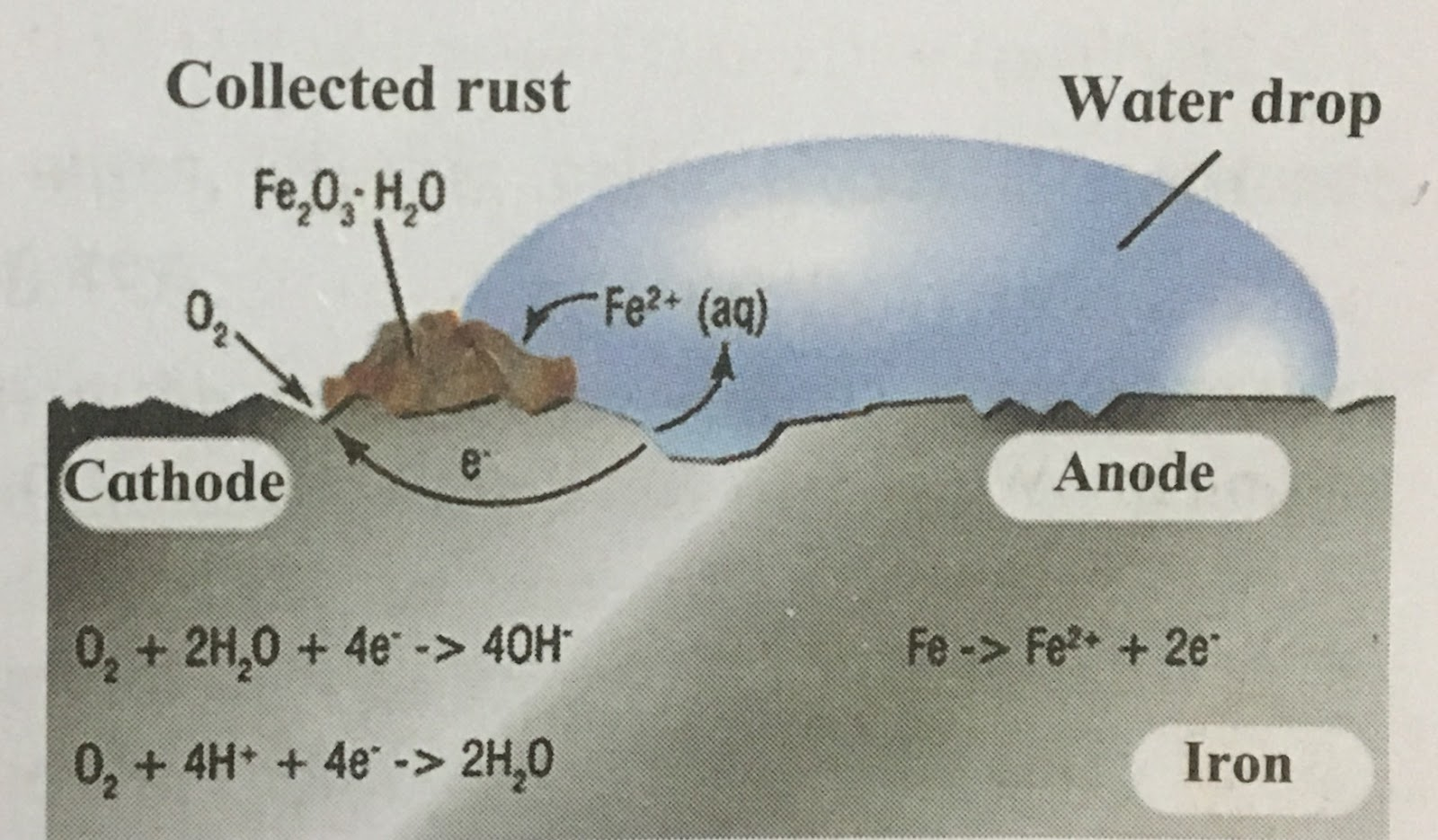### Chemical Reactions and Equations

Chemical Reactions and Equations

1. Choose the correct option from the bracket and explain the statement giving reason.

(Oxidation, displacement, electrolysis, reduction, zinc, copper, double displacement, decomposition)

a. To prevent rusting, a layer of ______________ metal is applied on iron sheets.

Ans. To prevent rusting,  a layer of zinc metal is applied on iron sheets.

Explanation: Zinc is a non-corrosive metal. Therefore, layer of zinc prevents rusting.

b. The conversion of ferrous sulphate to ferric sulphate is ____________ reaction.

Ans. The conversion of ferrous sulphate to ferric sulphate is oxidation reaction.

Explanation: Oxidation means losing one or more electrons. During this conversion, Fe2+ is converted into Fe3+ by losing one electron. Therefore, it is an oxidation reaction.

c. When electric current is passed through acidulated water ____________ of water takes place.

Ans. When electric current is passed through acidulated water decomposition of water takes place.

Explanation: Water decomposes into hydrogen and oxygen on passing of electric current through acidulated water. Therefore, it is a decomposition reaction.

d. Addition of an aqueous solution of ZnSO4 to an aqueous solution of BaCl2 is an example of ____________ reaction.

Ans. Addition of an aqueous solution of ZnSO4 to an aqueous solution of BaCl2 is an example of double displacement reaction.

Explanation: In this reaction, the iron in the reactants are exchanged to form a precipitate of BaSO4, Therefore, it is a double - displacement reaction.

2. Write answer to the following:

a. What is the reaction called when oxidation and reduction take place simultaneously? Explain with one example.

b. How can the rate of the chemical reaction, namely, decomposition of hydrogen peroxide be increased?

c. Explain the term reactant and product giving examples.

d. Explain the types of reaction with reference to oxygen and hydrogen. Illustrate with examples.

e. Explain the similarity and difference in two events, namely adding NaOH to water and adding CaO to water.

3. Explain the following terms with examples.

a. Endothermic reaction.
B. Combination reaction
C. Balanced equation
D. Displacement reaction.

4. Give Scientific reasons

a. When the gas formed on heating limestone is passed through freshly prepared lime water, the lime water turns milky.

B. It takes time for pieces of Shahabad tile to disappear in HCl, but its powder disappears rapidly.

C. While preparing dilute sulphuric acid from concentrated sulphuric acid in the laboratory, the concentrated sulphuric acid is added slowly to water with constant stirring.

D. It is recommended to use air tight container for storing oil for long time.

5. Observe the following picture and write down the chemical reaction with explanation.6. Identify the following reactions the reactants that undergo oxidation and reduction.

7. Balance the following equation stepwise.

8. Identify the endothermic and exothermic reaction.

9. Match the column in the following table.

Project

Prepare aqueous solutions of various solid salts available in the laboratory. Observe what happens when aqueous solution of sodium hydroxide is added to these. Prepare a chart of double displacement reactions based on these observation.